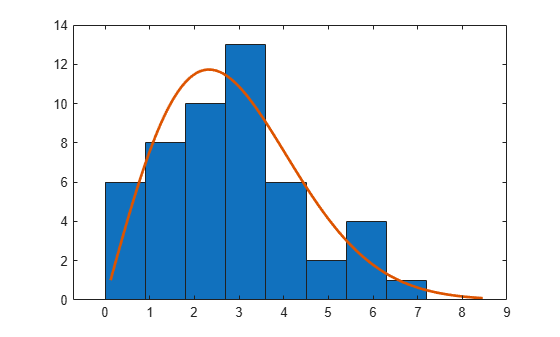# raylrnd

Rayleigh random numbers

## Syntax

``r = raylrnd(b)``
``r = raylrnd(b,sz1,...,szN)``
``r = raylrnd(b,sz)``

## Description

example

````r = raylrnd(b)` generates a random number from each Rayleigh distribution specified by the scale parameter `b`. You can specify `b` as a scalar, vector, matrix, or multidimensional array.```

example

````r = raylrnd(b,sz1,...,szN)` generates an array of Rayleigh random numbers, where `sz1,...,szN` indicates the size of each dimension.```

example

````r = raylrnd(b,sz)` generates an array of Rayleigh random numbers, where vector `sz` specifies `size(r)`.```

## Examples

collapse all

Generate random numbers from multiple Rayleigh distributions.

Generate a single random number from the Rayleigh distribution with scale parameter 6.

`r = raylrnd(6)`
```r = 11.4665 ```

Generate a vector of random numbers from Rayleigh distributions with different scale parameters.

```b = [6 4 1]; r = raylrnd(b)```
```r = 1×3 15.6604 3.8602 0.4680 ```

Generate a vector of random numbers drawn from the Rayleigh distribution with scale parameter 2.

`r = raylrnd(2,50,1)`
```r = 50×1 2.0347 3.6710 5.1289 2.8165 0.6377 4.0294 1.7668 1.0106 7.1710 5.9727 ⋮ ```

Plot a histogram of the result with a probability density function.

`histfit(r,8,"rayleigh")`Generate a 4-by-3-by-2 multidimensional array of random numbers from the Rayleigh distribution with scale parameter 0.5.

`raylrnd(0.5,[4 3 2])`
```ans = ans(:,:,1) = 0.3634 0.2168 1.8673 1.0528 0.7632 1.4427 1.1865 0.4943 1.6195 0.4570 0.5986 1.6793 ans(:,:,2) = 0.3975 0.0804 0.5475 0.3788 0.7546 0.6039 0.7728 0.7224 0.3680 0.8619 0.7257 0.8735 ```

## Input Arguments

collapse all

Scale parameter of the Rayleigh distribution, specified as a positive scalar value or an array of positive scalar values.

To generate random numbers from multiple distributions, specify `b` using an array. If `b` is a scalar, then `raylrnd `expands the scalar argument into a constant array whose size matches the dimensions `sz1,...,szN` or `sz`. Each element in `r` is a random number generated from the distribution specified by the corresponding element in `b`.

Example: `2.5`

Example: `[3 4 5]`

Data Types: `single` | `double`

Size of each dimension, specified as separate arguments of integers. If `b` is an array, then the specified dimensions `sz1,...,szN` must match the dimensions of `b`. The default values of `sz1,...,szN` are the dimensions of `b`.

• If you specify a single value `sz1`, then `r` is a square matrix of size `sz1`-by-`sz1`.

• If the size of any dimension is 0 or negative, then `r` is an empty array.

• Beyond the second dimension, `raylrnd` ignores trailing dimensions with a size of 1. For example, `raylrnd(0.4,1,4,1,1,1,1)` produces a 1-by-4 vector of random numbers from the Rayleigh distribution with scale 0.4.

Example: `7,6`

Data Types: `single` | `double`

Size of each dimension, specified as a row vector of integers. If `b` is an array, then the specified dimensions `sz` must match the dimensions of `b`. The default values of `sz` are the dimensions of `b`.

• If you specify a single value `[sz1]`, then `r` is a square matrix of size `sz1`-by-`sz1`.

• If the size of any dimension is 0 or negative, then `r` is an empty array.

• Beyond the second dimension, `raylrnd` ignores any trailing dimensions with a size of 1. For example, ```raylrnd(0.4,[1 4 1 1 1 1])``` produces a 1-by-4 vector of random numbers from the Rayleigh distribution with scale 0.4.

Example: `[7 6]`

Data Types: `single` | `double`

## Output Arguments

collapse all

Rayleigh random numbers, returned as a nonnegative scalar value or an array of nonnegative scalar values with the dimensions determined by `b`, `sz1,...,szN`, or `sz`. Each element in `r` is a random number generated from the distribution specified by the corresponding scale parameter in `b`.

## Alternative Functionality

• `raylrnd` is a function specific to the Rayleigh distribution. Statistics and Machine Learning Toolbox™ also offers the generic function `random`, which supports various probability distributions. To use `random`, create a `RayleighDistribution` probability distribution object and pass the object as an input argument or specify the probability distribution name and its parameter. Note that the distribution-specific function `raylrnd` is faster than the generic function `random`.

• To generate random numbers interactively, use `randtool`, a user interface for random number generation.

## Version History

Introduced before R2006a